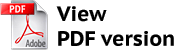https://vestnik.volbi.ru/
﻿

# Problems of application of the weighted average capital cost method for evaluation of the power project discount rate

Back to articles of current journal
 PDF:Author(s): Belchikova E. S., Сhеrnоv S. S., Number of journal: 4(25) Date: November 2013 Annotation: The Russian Federation energy industries current state and the necessity of the new investment projects establishment in this sector are considered in the article. The basic approaches to the discount rate definition are described and possibilities of their use in the energy projects calculation are analyzed. The recommendations for improvement of the weighted average cost of capital method as the most appropriate method for estimating the discount rate of energy project by taking into account in the calculation formula of accounts payable are presented. The recommendations for the estimation of the cost of accounts payable for calculation of the weighted average cost of capital are introduced. Keywords: economic evaluation of investments, investment projects in the energy sector, discount rate, accounts payable, accounts payable cost, measure of systematic risk, risk-free rate of assets return, average market rate of return, cumulative construction method, balance sheet of energy companies For citation: